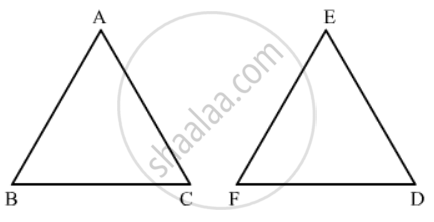Advertisement Remove all ads

# If in Two Triangle Abc and Def, ∠A = ∠E, ∠B = ∠F, Then Which of the Following is Not True? (A) B C D F = a C D E (B) a B D E = B C D F (C) a B E F = a C D E (D) B C D F = a B E F - Mathematics

MCQ

If in two triangle ABC and DEF, ∠A = ∠E, ∠B = ∠F, then which of the following is not true?

(a)$\frac{BC}{DF} = \frac{AC}{DE}$

(b)$\frac{AB}{DE} = \frac{BC}{DF}$

(c)$\frac{AB}{EF} = \frac{AC}{DE}$

(d)$\frac{BC}{DF} = \frac{AB}{EF}$

#### Options

• $\frac{BC}{DF} = \frac{AC}{DE}$
• $\frac{AB}{DE} = \frac{BC}{DF}$
• $\frac{AB}{EF} = \frac{AC}{DE}$
• $\frac{BC}{DF} = \frac{AB}{EF}$
Advertisement Remove all ads

#### SolutionIn ΔABC and ΔDEF

∠ A = ∠ E

∠ B = ∠ F

∴ ΔABC and ΔDEF are similar triangles.

Hence (AB)/(EF)=(BC)/(FD)=(CA)/(DE)

Hence the correct answer is (b).

Concept: Triangles Examples and Solutions
Is there an error in this question or solution?
Advertisement Remove all ads

#### APPEARS IN

RD Sharma Class 10 Maths
Chapter 7 Triangles
Q 31 | Page 133
Advertisement Remove all ads

#### Video TutorialsVIEW ALL 

Advertisement Remove all ads
Share
Notifications

View all notifications

Forgot password?
Course